# Support Vector Machines

#### 3/16/2020

# load python
library(reticulate)
use_python('C:/Users/Andrew/Anaconda3/')
library(knitr)
# load packages
import numpy as np
import matplotlib.pyplot as plt
import seaborn as sns; sns.set()

from sklearn.datasets import make_blobs, fetch_openml, make_moons
from sklearn.svm import SVC, LinearSVC
from sklearn.preprocessing import StandardScaler
from sklearn import metrics
from sklearn.model_selection import train_test_split
from sklearn.multiclass import OneVsRestClassifier
from sklearn.model_selection import RandomizedSearchCV
from scipy.stats import reciprocal, uniform
from sklearn.decomposition import PCA
from sklearn.pipeline import make_pipeline

np.random.seed(0) # set seed

# 1 Introduction

The Support Vector Machine (SVM) algorithm is part of a family of classifier and regression algorithms that aim to predict the class or value of an observation. The SVM algorithm identifies data points, called support vectors, that generate the widest possible margin between two classes in order to yield the best classification generalization. The SVM is made powerful by the use of kernels, a function that computes the dot product of two vectors, thereby allowing us to effectively skip feature transformations and consequently improve computation performance.

# 2 When to Use SVMs

SVMs run exceptionally well on a variety of data sets and we can use SVMs to perform linear or nonlinear classification to include outlier detection. When running SVMs, we need to consider: (1) the kernel, and (2) regularization hyperparameters of C and $$\gamma$$. We can also use SVMs for linear and nonlinear regression through the absence or presence of kernels. SVMs are also used to produce rank order problems as well.

# 3 Kernels

While there are many kernels to choose from, SVMs are most commonly employed with either no kernel, thereby making it a linear SVM, or a Gaussian kernel. We might consider using a linear SVM when we have many independent variables and a small set of observations for our training data. Alternatively, we might consider using a Gaussian kernel when we have few independent variables but many observations for our training data. There are other kernels, like the Polynomial kernel which generates polynomials of the independent variables, which is at times used to produce a linearly separable data set from a non linear set of data. The string kernel is used when our independent variables are words and when we want to measure the distance between two similar spellings of words.

# 4 Quantity of Interests: Support Vectors

To derive our quantity of interest and to observe the intuition behind SVMs, we use sklearn to derive the support vectors for our first linear SVM. We begin by generating some data:

X, y = make_blobs(n_samples = 50, centers = 2,
random_state = 0, cluster_std = 0.60)

Next, we fit a linear support vector classifier to the data:

# fit a linear SVC with a very low regularization and probability = True
model = SVC(kernel='linear', C = 1E10, probability = True)
model.fit(X, y)
## SVC(C=10000000000.0, break_ties=False, cache_size=200, class_weight=None,
##     coef0=0.0, decision_function_shape='ovr', degree=3, gamma='scale',
##     kernel='linear', max_iter=-1, probability=True, random_state=None,
##     shrinking=True, tol=0.001, verbose=False)

Then, we prepare a function that plots the decision function for a bivariate SVM.

def plot_svc_decision_function(model):
''' This function plots the decision function of a bivariate SVM
in the following steps. First, we create an empty graph. Second,
we create a matrix of points to calculate the distance of our observations
from the decision boundary. Third, we plot our decision boundary.'''

ax = plt.gca() # create empty plot
xlim = ax.get_xlim() # xlim tuple
ylim = ax.get_ylim() # ylim tuple

# create grid to evaluate model
x = np.linspace(xlim, xlim, 30) # create 30 values between 0 and 1
y = np.linspace(ylim, ylim, 30) # create 30 values between 0 and 1

# returns two 2-D matrix representing the X and Y coordinates of all the points
X, Y = np.meshgrid(x, y)

# stack x-y into 2-D matrix; 900 rows, 2 cols
xy = np.vstack([X.ravel(), Y.ravel()]).T

# pass points into decision_function; return signed distance from observation to hyperplane
P = model.decision_function(xy).reshape(X.shape)

# plot decision boundary
ax.contour(X, Y, P, colors='k',
levels=[-1, 0, 1], alpha=0.5,
linestyles=['--', '-', '--'])

# plot support vectors
ax.scatter(model.support_vectors_[:, 0],
model.support_vectors_[:, 1],
s=300, linewidth=1, facecolors='none');
ax.set_xlim(xlim)
ax.set_ylim(ylim)

The graph below plots our synthetic data as well as the SVM’s boundary and margins. The dashed lines run through the three support vectors while the solid line represents the hyperplane that maximizes the margin between the two classes; thereby linearly separating the data.

# plot svm boundaries
plt.scatter(X[:, 0], X[:, 1], c=y, s=50, cmap='autumn')
plot_svc_decision_function(model);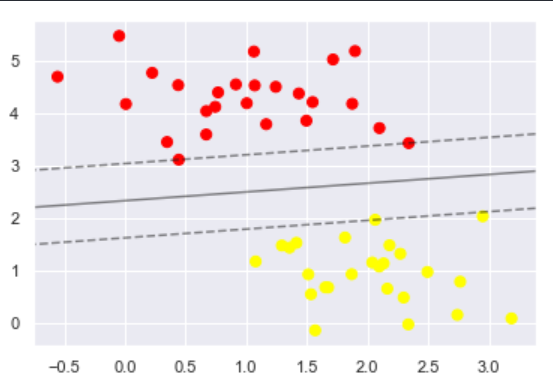Visualizing the Data

To identify our support vector quantities of interest, we can use the support_vectors_ attribute. Notice that the x and y coordinates for the support vectors match the graph above.

print(model.support_vectors_)
## [[0.44359863 3.11530945]
##  [2.33812285 3.43116792]
##  [2.06156753 1.96918596]]

# 5 Quantity of Interest: Distance from Separating Hyperplane

Next, we can view how far our data points lie, as measured by the signed distance from the hyperplane margin by executing:

model.decision_function(X)[:5] # view first 5
## array([ 1.46710653,  1.41896247, -2.54881397, -2.16834939,  2.26494334])

# 6 Quantity of Interest: Predicted Class Probability

Lastly, because we specified probability = True in our model, we can also derive the predicted probabilities for the class of each observation by using the predict_proba attribute.

model.predict_proba(X)[:5] # view first 5
## array([[0.10383175, 0.89616825],
##        [0.11053993, 0.88946007],
##        [0.97506858, 0.02493142],
##        [0.95687944, 0.04312056],
##        [0.03505638, 0.96494362]])

# 7 Hyperparameter of Interest: C (Inverse Regularization)

C is one the first primary hyperparameters that we need to specify, and tweak, when running a SVM. C is related to the idea of regularization which aims to constrain the model such that it can generalize better to unseen data. Put differently, we regularize our model by reducing C to limit overfitting. A more generalizable model is one that uses a low C value while a model that imposes harder margins is one that uses high values of C. We demonstrate this fact below:

X, y = make_blobs(n_samples = 400, centers = 2,
random_state = 0, cluster_std = 1.00)

model = SVC(kernel='linear', C = 1, probability = True)
model.fit(X, y)

plt.scatter(X[:, 0], X[:, 1], c=y, s=50, cmap='autumn')
plot_svc_decision_function(model);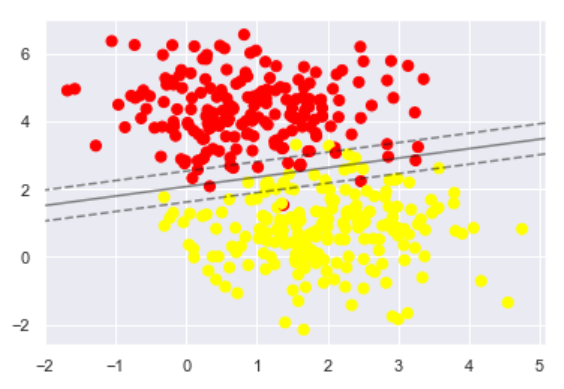Low Regularization

model = SVC(kernel='linear', C = 1E6, probability = True)
model.fit(X, y)

plt.scatter(X[:, 0], X[:, 1], c=y, s=50, cmap='autumn')
plot_svc_decision_function(model);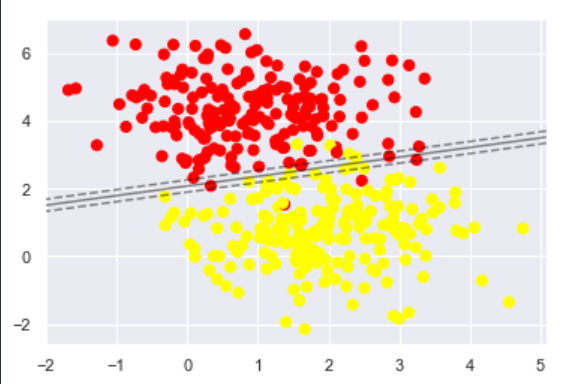High Regularization

# 8 Hyperparameter of Interest: $$\gamma$$

$$\gamma$$ is a hyperparameter that becomes accessible when we use a Gaussian model; the default state of sklearn’s SVC. As $$\gamma$$ increases, the decision boundary becomes more irregular by fitting a more narrow bell-shaped curve around the data. As $$\gamma$$ decreases, the decision boundary becomes more smooth by fitting a wider bell-shaped curve around the data. For graphical intuition behind $$\gamma$$ and its relationship with C, we draw on Géron’s work, as shown below:1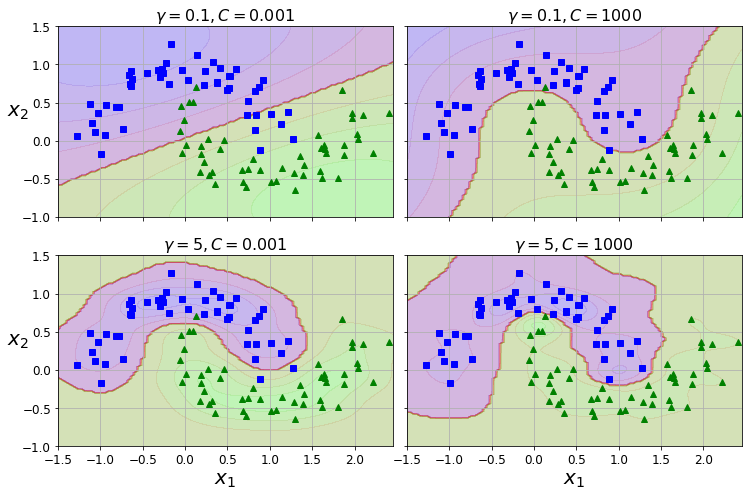Regularizations: $$\gamma$$ and C

# 9 SVMs in Practice

## 9.1 Gaussian SVM

To demonstrate the use of SVMs in practice, we begin with some synthetic toy examples to draw on the use of the Gaussian model followed by a linear model based on MNIST.

We begin by generating some new data using sklearn’s make_blobs:

X, y = make_blobs(n_samples = 1000, centers = 4,
random_state = 0, cluster_std = 1.03)

plt.scatter(X[:, 0], X[:, 1], c = y, s = 50, cmap = 'autumn')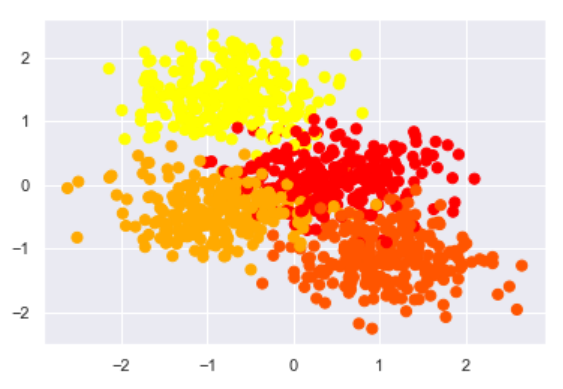Visualizing the Data

We begin by scaling and splitting our data.

# scale data
scaler = StandardScaler()
X = scaler.fit_transform(X)

# split data
Xtrain, Xtest, ytrain, ytest = train_test_split(X, y, random_state = 42)

Next, we fit our SVC model by attaching the Gaussian kernel and setting its C and $$\gamma$$, which was chosen ex-post following a random grid search.

# fit model
model = OneVsRestClassifier(SVC(kernel = 'rbf', C = 1.9, gamma = 1.1))
model.fit(Xtrain, ytrain)
## OneVsRestClassifier(estimator=SVC(C=1.9, break_ties=False, cache_size=200,
##                                   class_weight=None, coef0=0.0,
##                                   decision_function_shape='ovr', degree=3,
##                                   gamma=1.1, kernel='rbf', max_iter=-1,
##                                   probability=False, random_state=None,
##                                   shrinking=True, tol=0.001, verbose=False),
##                     n_jobs=None)

To see how we did, we generate some results like so:

# generate results
predicted_y = model.predict(Xtest) # predict labels from test X
f1 = metrics.f1_score(ytest, predicted_y, average="weighted") # prepare f1
correct_pred = predicted_y == ytest # manual accuracy for fun

# compare actual to predicted
total = len(ytest)
correct = 0.0
accuracy = 0.0
for j in range(len(ytest)):
if correct_pred[j]: # if true
correct += 1 #  add 1
accuracy = correct / total # generate accuracy
print('the accuracy is ', accuracy)
## the accuracy is  1.0
print('the f1 is ', f1)
## the f1 is  1.0

Since we have two hyperparameters, C and $$\gamma$$, we use a randomized grid search to look for the best performing model.

# grid search
param_distributions = {"estimator__gamma": reciprocal(0.001, 10), "estimator__C": uniform(0.001, 10)}
rnd_search_cv = RandomizedSearchCV(model, param_distributions, n_iter = 10, verbose = 2, cv = 3)
rnd_search_cv.fit(Xtrain, ytrain)
## Fitting 3 folds for each of 10 candidates, totalling 30 fits
## [CV] estimator__C=7.152893663724195, estimator__gamma=0.25766385746135884
## [CV]  estimator__C=7.152893663724195, estimator__gamma=0.25766385746135884, total=   0.0s
## [CV] estimator__C=7.152893663724195, estimator__gamma=0.25766385746135884
## [CV]  estimator__C=7.152893663724195, estimator__gamma=0.25766385746135884, total=   0.0s
## [CV] estimator__C=7.152893663724195, estimator__gamma=0.25766385746135884
## [CV]  estimator__C=7.152893663724195, estimator__gamma=0.25766385746135884, total=   0.0s
## [CV] estimator__C=5.449831829968969, estimator__gamma=0.04950159553733192
## [CV]  estimator__C=5.449831829968969, estimator__gamma=0.04950159553733192, total=   0.0s
## [CV] estimator__C=5.449831829968969, estimator__gamma=0.04950159553733192
## [CV]  estimator__C=5.449831829968969, estimator__gamma=0.04950159553733192, total=   0.0s
## [CV] estimator__C=5.449831829968969, estimator__gamma=0.04950159553733192
## [CV]  estimator__C=5.449831829968969, estimator__gamma=0.04950159553733192, total=   0.0s
## [CV] estimator__C=6.459941130666562, estimator__gamma=0.05627932047415165
## [CV]  estimator__C=6.459941130666562, estimator__gamma=0.05627932047415165, total=   0.0s
## [CV] estimator__C=6.459941130666562, estimator__gamma=0.05627932047415165
## [CV]  estimator__C=6.459941130666562, estimator__gamma=0.05627932047415165, total=   0.0s
## [CV] estimator__C=6.459941130666562, estimator__gamma=0.05627932047415165
## [CV]  estimator__C=6.459941130666562, estimator__gamma=0.05627932047415165, total=   0.0s
## [CV] estimator__C=8.918730007820797, estimator__gamma=7.155682161754859
## [CV]  estimator__C=8.918730007820797, estimator__gamma=7.155682161754859, total=   0.0s
## [CV] estimator__C=8.918730007820797, estimator__gamma=7.155682161754859
## [CV]  estimator__C=8.918730007820797, estimator__gamma=7.155682161754859, total=   0.0s
## [CV] estimator__C=8.918730007820797, estimator__gamma=7.155682161754859
## [CV]  estimator__C=8.918730007820797, estimator__gamma=7.155682161754859, total=   0.0s
## [CV] estimator__C=3.835415188257777, estimator__gamma=1.4685885989200846
## [CV]  estimator__C=3.835415188257777, estimator__gamma=1.4685885989200846, total=   0.0s
## [CV] estimator__C=3.835415188257777, estimator__gamma=1.4685885989200846
## [CV]  estimator__C=3.835415188257777, estimator__gamma=1.4685885989200846, total=   0.0s
## [CV] estimator__C=3.835415188257777, estimator__gamma=1.4685885989200846
## [CV]  estimator__C=3.835415188257777, estimator__gamma=1.4685885989200846, total=   0.0s
## [CV] estimator__C=5.289949197529045, estimator__gamma=0.18714500686240662
## [CV]  estimator__C=5.289949197529045, estimator__gamma=0.18714500686240662, total=   0.0s
## [CV] estimator__C=5.289949197529045, estimator__gamma=0.18714500686240662
## [CV]  estimator__C=5.289949197529045, estimator__gamma=0.18714500686240662, total=   0.0s
## [CV] estimator__C=5.289949197529045, estimator__gamma=0.18714500686240662
## [CV]  estimator__C=5.289949197529045, estimator__gamma=0.18714500686240662, total=   0.0s
## [CV] estimator__C=9.25696638292661, estimator__gamma=0.001923730509654649
## [CV]  estimator__C=9.25696638292661, estimator__gamma=0.001923730509654649, total=   0.0s
## [CV] estimator__C=9.25696638292661, estimator__gamma=0.001923730509654649
## [CV]  estimator__C=9.25696638292661, estimator__gamma=0.001923730509654649, total=   0.0s
## [CV] estimator__C=9.25696638292661, estimator__gamma=0.001923730509654649
## [CV]  estimator__C=9.25696638292661, estimator__gamma=0.001923730509654649, total=   0.0s
## [CV] estimator__C=0.8722929970154071, estimator__gamma=0.001204685241203032
## [CV]  estimator__C=0.8722929970154071, estimator__gamma=0.001204685241203032, total=   0.0s
## [CV] estimator__C=0.8722929970154071, estimator__gamma=0.001204685241203032
## [CV]  estimator__C=0.8722929970154071, estimator__gamma=0.001204685241203032, total=   0.0s
## [CV] estimator__C=0.8722929970154071, estimator__gamma=0.001204685241203032
## [CV]  estimator__C=0.8722929970154071, estimator__gamma=0.001204685241203032, total=   0.0s
## [CV] estimator__C=8.32719845547938, estimator__gamma=1.2960656597279723
## [CV]  estimator__C=8.32719845547938, estimator__gamma=1.2960656597279723, total=   0.0s
## [CV] estimator__C=8.32719845547938, estimator__gamma=1.2960656597279723
## [CV]  estimator__C=8.32719845547938, estimator__gamma=1.2960656597279723, total=   0.0s
## [CV] estimator__C=8.32719845547938, estimator__gamma=1.2960656597279723
## [CV]  estimator__C=8.32719845547938, estimator__gamma=1.2960656597279723, total=   0.0s
## [CV] estimator__C=8.70112148246819, estimator__gamma=8.212461922256862
## [CV]  estimator__C=8.70112148246819, estimator__gamma=8.212461922256862, total=   0.0s
## [CV] estimator__C=8.70112148246819, estimator__gamma=8.212461922256862
## [CV]  estimator__C=8.70112148246819, estimator__gamma=8.212461922256862, total=   0.0s
## [CV] estimator__C=8.70112148246819, estimator__gamma=8.212461922256862
## [CV]  estimator__C=8.70112148246819, estimator__gamma=8.212461922256862, total=   0.0s
## RandomizedSearchCV(cv=3, error_score=nan,
##                    estimator=OneVsRestClassifier(estimator=SVC(C=1.9,
##                                                                break_ties=False,
##                                                                cache_size=200,
##                                                                class_weight=None,
##                                                                coef0=0.0,
##                                                                decision_function_shape='ovr',
##                                                                degree=3,
##                                                                gamma=1.1,
##                                                                kernel='rbf',
##                                                                max_iter=-1,
##                                                                probability=False,
##                                                                random_state=None,
##                                                                shrinking=True,
##                                                                tol=0.001,
##                                                                verbose=False),
##                                                  n_jobs=None),
##                    iid='deprecated', n_iter=10, n_jobs=None,
##                    param_distributions={'estimator__C': <scipy.stats._distn_infrastructure.rv_frozen object at 0x0000000020E13A20>,
##                                         'estimator__gamma': <scipy.stats._distn_infrastructure.rv_frozen object at 0x0000000020E13710>},
##                    pre_dispatch='2*n_jobs', random_state=None, refit=True,
##                    return_train_score=False, scoring=None, verbose=2)
##
## [Parallel(n_jobs=1)]: Using backend SequentialBackend with 1 concurrent workers.
## [Parallel(n_jobs=1)]: Done   1 out of   1 | elapsed:    0.0s remaining:    0.0s
## [Parallel(n_jobs=1)]: Done  30 out of  30 | elapsed:    0.0s finished

We then use the best_estimator_ and best_score_ attributes to review the best performing hyperparameters and its average cross-validated score.

print(rnd_search_cv.best_estimator_) # estimator that was chosen by the search
## OneVsRestClassifier(estimator=SVC(C=7.152893663724195, break_ties=False,
##                                   cache_size=200, class_weight=None, coef0=0.0,
##                                   decision_function_shape='ovr', degree=3,
##                                   gamma=0.25766385746135884, kernel='rbf',
##                                   max_iter=-1, probability=False,
##                                   random_state=None, shrinking=True, tol=0.001,
##                                   verbose=False),
##                     n_jobs=None)
print(rnd_search_cv.best_score_) # mean cross-validated score of the best_estimator
## 1.0

## 9.2 Linear SVM

MNIST (Modified National Institute of Standards and Technology database) is a famous data set used to formulate and test new algorithms among researchers and applied practitioners. In the chunk below, we begin by loading our data using sklearn’s handy package to access MNIST. We then rescale the X array between 0 and 1 by dividing by its maximum and then we shuffle the data and establish some training and test sets.

# load MNSIT from https://www.openml.org/d/554 via fetch_openml
X, Y = fetch_openml(name='mnist_784', return_X_y=True, cache=False)

# rescale grayscale values to [0,1].
X = X / 255.0

# shuffle the data
shuffle = np.random.permutation(np.arange(X.shape)) # generate unique random indices
X, Y = X[shuffle], Y[shuffle] # apply shuffle

# prepare some datasets
test_data, test_labels = X[61000:], Y[61000:]
dev_data, dev_labels = X[60000:61000], Y[60000:61000]
train_data, train_labels = X[:60000], Y[:60000]
mini_train_data, mini_train_labels = X[:5000], Y[:5000]

# split data
Xtrain, Xtest, ytrain, ytest = train_test_split(mini_train_data, mini_train_labels,
random_state=42)

### 9.2.1 Visualize the Data

Since MNIST is a data set of handwritten digits, we can visualize the data thanks to imshow from matplotlib. In the chunk below, we collect 10 examples of all 10 digits and display them in a 2x2 matrix.

def visualize_mnist(label_array):
fig, ax = plt.subplots(10, 10, figsize = (10,10))  # create 10x10 subplots

for i in range(0, 10): # initiate loop
find_digit = np.where(label_array == str(i)) # find numbers 0-9
find_digit = mini_train_data[find_digit][0:10] # truncate data

for j in range(0, 10): # initiate loop
working_item = find_digit[j] # pull individual arrays
working_item.shape = (28, 28) # reshape for viewing
working_item = 255 - working_item # subtract itself for white background
ax[i, j].imshow(working_item, cmap = 'gray') # plot the image
ax[i, j].set_xticks([]) # remove axis ticks for clarity
ax[i, j].set_yticks([]) # remove axis ticks for clarity

return plt.show()

visualize_mnist(mini_train_labels)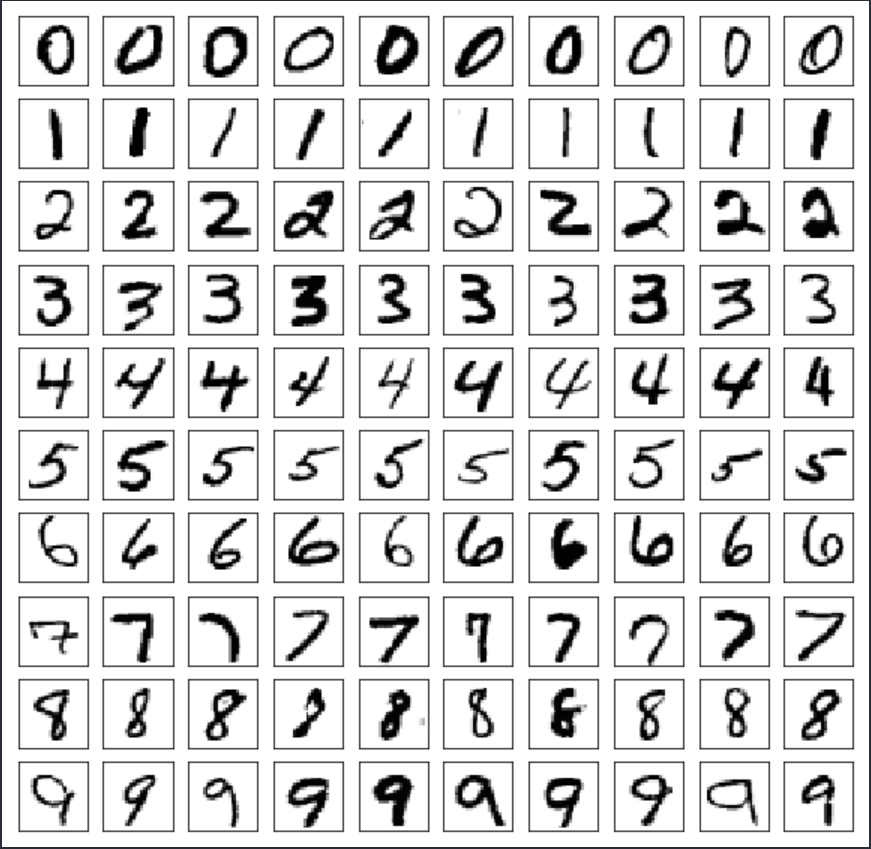Visualizing the Data

### 9.2.2 An Initial Linear SVM on MNIST

Since SVM classifiers are binary classifiers, we need to remember to use OneVsRest to classify all of our digits. However, since we are beginning with a linear SVM classifier, the model will automatically force OneVsRest. Again, remember what we are trying to model here. Our independent variables are 28x28 pixel images; meaning they are 784 dimensional. Our dependent variables are digit labels, ranging from 0 to 9.

# fit model
model = LinearSVC(random_state = 42, tol = 0.1)
model.fit(Xtrain, ytrain)
## LinearSVC(C=1.0, class_weight=None, dual=True, fit_intercept=True,
##           intercept_scaling=1, loss='squared_hinge', max_iter=1000,
##           multi_class='ovr', penalty='l2', random_state=42, tol=0.1, verbose=0)

Next, we generate our quantities of interest: accuracy and f1 score.

# generate results
predicted_y = model.predict(Xtest) # predict labels from test X
f1 = metrics.f1_score(ytest, predicted_y, average="weighted") # prepare f1
correct_pred = predicted_y == ytest # manual accuracy for fun

# compare actual to predicted
total = len(ytest)
correct = 0.0
accuracy = 0.0
for j in range(len(ytest)):
if correct_pred[j]: # if true
correct += 1 #  add 1
accuracy = correct / total # generate accuracy
print('the accuracy is ', accuracy)
## the accuracy is  0.8472
print('the f1 is ', f1)
## the f1 is  0.8467613978590314

While 84% accuracy is not the greatest, it serves as a useful baseline metric for comparison. Next, we unleash the power of the SVC by using a kernel. The hyperparameters were chosen from random grid searches, ex-post.

### 9.2.3 Gaussian SVM

# fit model
model = SVC(kernel='rbf', C = 8.81, gamma = 0.02, random_state = 42)
model.fit(Xtrain, ytrain)
## SVC(C=8.81, break_ties=False, cache_size=200, class_weight=None, coef0=0.0,
##     decision_function_shape='ovr', degree=3, gamma=0.02, kernel='rbf',
##     max_iter=-1, probability=False, random_state=42, shrinking=True, tol=0.001,
##     verbose=False)
# generate results
predicted_y = model.predict(Xtest) # predict labels from test X
f1 = metrics.f1_score(ytest, predicted_y, average="weighted") # prepare f1
correct_pred = predicted_y == ytest # manual accuracy for fun

# compare actual to predicted
total = len(ytest)
correct = 0.0
accuracy = 0.0
for j in range(len(ytest)):
if correct_pred[j]: # if true
correct += 1 #  add 1
accuracy = correct / total # generate accuracy
print('the accuracy is ', accuracy)
## the accuracy is  0.9488
print('the f1 is ', f1)
## the f1 is  0.9486102450429073

We can see that the use of the kernel and improved hyperparameter tuning improved our predictive capability by 10%.

### 9.2.4 PCA: Gaussian SVM

Lastly, since we are working with very high dimensional data, we can use principal component analysis, an unsupervised algorithm, to speed up computation by reducing the number of features we are analyzing. Using PCA for dimensionality reduction involves zeroing out one or more of the smallest principal components, resulting in a lower-dimensional projection of the data that preserves the maximal data variance.

We begin by specifying our PCA, fitting our Gaussian SVC (whose parameters were again chosen ex-post following a randomized grid search), and then we pipeline and fit our model.

pca = PCA(n_components = 28, svd_solver = 'randomized', random_state = 42)
svc = SVC(kernel='rbf', C = 4.13, gamma = 0.07, random_state = 42, class_weight='balanced')
model = make_pipeline(pca, svc)
model.fit(Xtrain, ytrain)
## Pipeline(memory=None,
##          steps=[('pca',
##                  PCA(copy=True, iterated_power='auto', n_components=28,
##                      random_state=42, svd_solver='randomized', tol=0.0,
##                      whiten=False)),
##                 ('svc',
##                  SVC(C=4.13, break_ties=False, cache_size=200,
##                      class_weight='balanced', coef0=0.0,
##                      decision_function_shape='ovr', degree=3, gamma=0.07,
##                      kernel='rbf', max_iter=-1, probability=False,
##                      random_state=42, shrinking=True, tol=0.001,
##                      verbose=False))],
##          verbose=False)

Next, we generate our prediction quantities of interest as usual:

# generate results
predicted_y = model.predict(Xtest) # predict labels from test X
f1 = metrics.f1_score(ytest, predicted_y, average="weighted") # prepare f1
correct_pred = predicted_y == ytest # manual accuracy for fun

# compare actual to predicted
total = len(ytest)
correct = 0.0
accuracy = 0.0
for j in range(len(ytest)):
if correct_pred[j]: # if true
correct += 1 #  add 1
accuracy = correct / total # generate accuracy
print('the accuracy is ', accuracy)
## the accuracy is  0.9544
print('the f1 is ', f1)
## the f1 is  0.9540938886070337

And we find that after using PCA, thereby reducing 784 dimensions to 28, we gain very similar results at a fraction of the time-cost. This is of course because many dimensions associated with the MNIST data set have little variation, as many pixels are simply empty.

# 10 Sources

• Matthias Feurer, Jan N. van Rijn, Arlind Kadra, Pieter Gijsbers, Neeratyoy Mallik, Sahithya Ravi, Andreas Mueller, Joaquin Vanschoren, Frank Hutter. OpenML-Python: an extensible Python API for OpenML. arXiv:1911.02490 [cs.LG], 2019

• LeCun, Yann. “The MNIST database of handwritten digits.” http://yann.lecun.com/exdb/mnist/ (1998).

• Géron, Aurélien. Hands-On Machine Learning with Scikit-Learn, Keras, and TensorFlow: Concepts, Tools, and Techniques to Build Intelligent Systems. O’Reilly Media, 2019.

1. Géron, Aurélien. Hands-On Machine Learning with Scikit-Learn, Keras, and TensorFlow: Concepts, Tools, and Techniques to Build Intelligent Systems. O’Reilly Media, 2019. https://github.com/ageron/handson-ml2/blob/master/05_support_vector_machines.ipynb